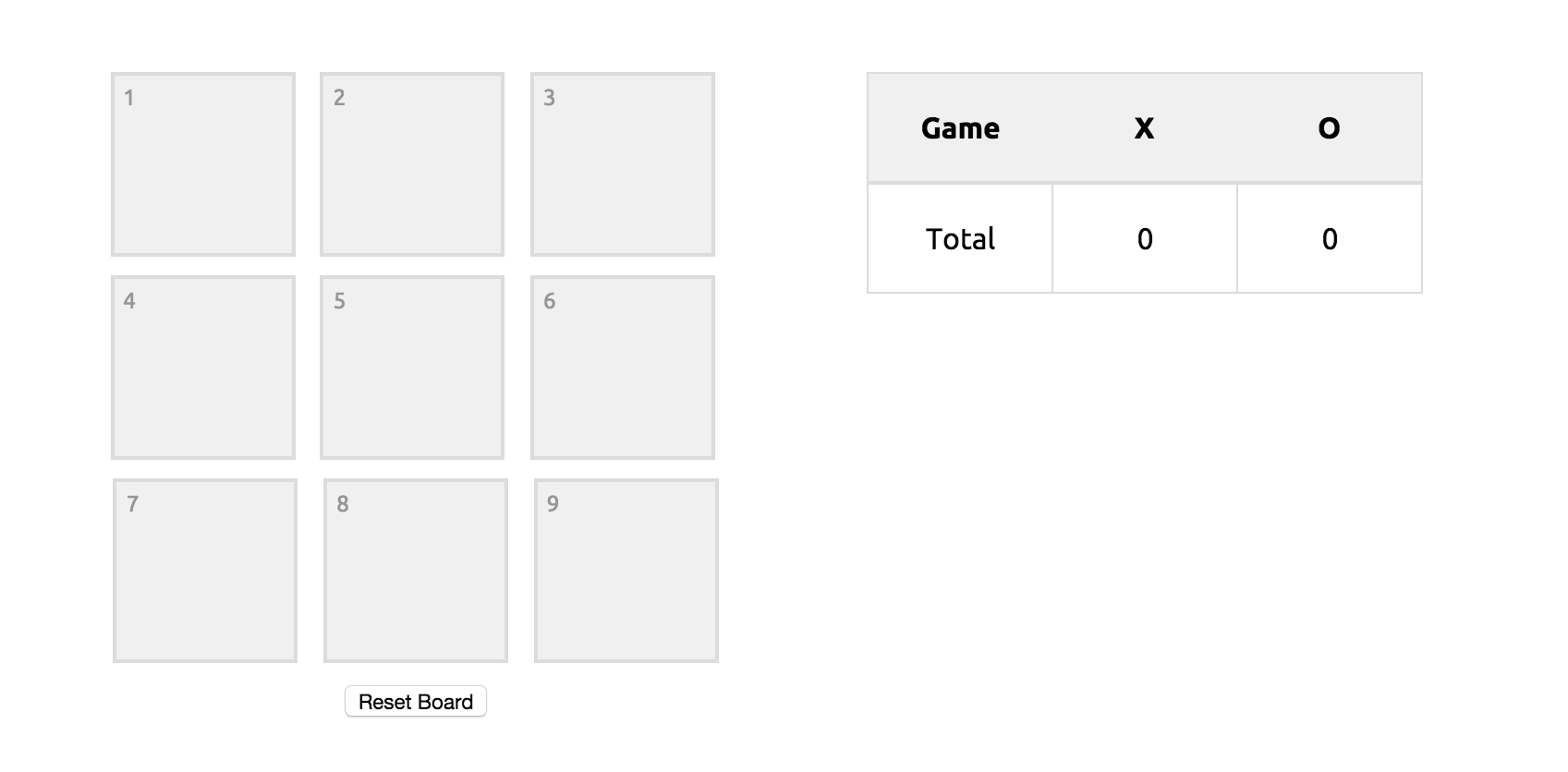# Tic Tac Toe (Revisited)

One of the first projects I did on this blog was a simple game of tic tac toe. It was one of my first attempts at building something in JavaScript that wasn’t purely for UI purposes. Looking back at it now, it is functional, but could definitely be improved in many ways. So this is how, 8 months later, I approached the project.## Project Overview #

The main problem with the way I handled the project the last time around was how I checked if each play was a winning play. Last time, I wrote out each possible win out by itself like this -

``if ( sq1.hasClass('X-play') && sq2.hasClass('X-play') && sq3.hasClass('X-play') ) {  winAlert("X");} else if ( sq1.hasClass('O-play') && sq2.hasClass('O-play') && sq3.hasClass('O-play') ) {  winAlert("O");}// repeat for every possible combination``

Old method

This was very tedious and repetitive.

This time around, I abstracted each of the winning combinations into an array of arrays, and wrote a function that would loop through each combination to determine if it was a winning one -

``// Winning combinationsvar wins = [  [1, 2, 3],  [4, 5, 6],  [7, 8, 9],  [1, 4, 7],  [2, 5, 8],  [3, 6, 9],  [1, 5, 9],  [3, 5, 7]];/* Register if winning combination has happened */function registerWin(x, y, z) {  if (    // All three winning squares played    \$('.square[data-square="'+x+'"]').data("played") === true &&    \$('.square[data-square="'+y+'"]').data("played") === true &&    \$('.square[data-square="'+z+'"]').data("played") === true    &&    // All three squares played by the same player    \$('.square[data-square="'+x+'"]').data("player") === \$('.square[data-square="'+y+'"]').data("player") &&    \$('.square[data-square="'+x+'"]').data("player") === \$('.square[data-square="'+z+'"]').data("player")  ) {    winner = \$('.square[data-square="'+x+'"]').data("player");    return true;  }} // end registerWin/* Check if there is a win */function checkWin() {  // Loop through all winning combinations  for (i = 0; i < wins.length; i++) {    var w = registerWin(wins[i], wins[i], wins[i]);    if (w) {      alert(winner+ " won!");      addWinToTable();      clearBoard();    }    if ( !w && i === (wins.length - 1) ) {      checkDraw();    }  } // end loop} // end checkWin``

Having the winning combinations abstracted in this way was also useful for making the program (playing as O) able to play tactically. I wrote a function that would look through the winning combinations to determine if any 2 of the 3 were played by the same player. If this was the case, then O should play the remaining square instead of something random.

``/* Funciton to check if 2 of 3 winning squares have been played by the same player */function checkTacticalPlay(x, y, z) {  // x and y played  if (    // 2 out of the 3 winning squares    \$('.square[data-square="'+x+'"]').data("played") === true &&    \$('.square[data-square="'+y+'"]').data("played") === true    &&    // Both squares played by the same player    \$('.square[data-square="'+x+'"]').data("player") === \$('.square[data-square="'+y+'"]').data("player")  ) {    // Return remaining square    return z;  }  // repeat for if x and z played  else if (    \$('.square[data-square="'+x+'"]').data("played") === true &&    \$('.square[data-square="'+z+'"]').data("played") === true    &&    \$('.square[data-square="'+x+'"]').data("player") === \$('.square[data-square="'+z+'"]').data("player")    ) { return y; }  // repeat for if z and y played  else if (    \$('.square[data-square="'+z+'"]').data("played") === true &&    \$('.square[data-square="'+y+'"]').data("played") === true    &&    \$('.square[data-square="'+z+'"]').data("player") === \$('.square[data-square="'+y+'"]').data("player")    ) { return x; }} // end checkTacticalPlay``

You can check out the full script for the game on my github repository or play the game here.

### Becoming More DRY #

What I've learned from trying to rewrite this is that, over the past 8 months, I have developed a better habit of trying to write more DRY code (Don't Repeat Yourself). This new method involves a lot less repetition and is therefore easier to maintain.

You never really notice how you've developed until you try to rewrite something you wrote before. If you have the time, I'd definitely suggest you give it a try (and let me know how you do)!

## Keep in touch KeepinTouch

### Subscribe to my Newsletter 📥

Receive quality articles and other exclusive content from myself. You’ll never receive any spam and can always unsubscribe easily.